# Explain the benefits of spread syntax & how it is different from rest syntax in ES6 ?

Spread Operator: Spread operator or Spread Syntax allow us to expand the arrays and objects into elements in the case of an array and key-value pairs in the case of an object. The spread syntax is represented by three dots (…) in JavaScript.

Syntax:

`var my_var = [...array];`

1. It allows us to include all elements of an array or object in a list of some kind. It can expand an object or an array and store all values in a new variable of the same datatype.

Example:

## JavaScript

 `<``script``>` `    ``// Operating spread syntax to expand an Object` `    ``var obj1 = {` `        ``organisation: "GFG",` `        ``fullForm: "Geeks for Geeks"` `    ``};` `    ``var obj2 = {` `        ``description: "A learning platform for Geeks."` `    ``};` `    ``var obj3 = {...obj2, ...obj1` `    ``};` `    ``console.log(obj3);` `    ``// Operating spread syntax to expand an Array` `    ``var fruits = ["Mango", "Banana", "Apple"];` `    ``var moreFruits = [...arr1, "Orange", ` `                ``"Pineapple", "Watermelon"];` `    ``console.log(moreFruits);` ``

Output: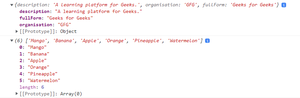Expanding an Array and an Object

2. It allows us to copy all elements of an already existing array into a new array and perform push, pop operations without even disturbing the previous array.

Example:

## JavaScript

 `<``script``>` `    ``// Copying an array into another ` `    ``// array and operate pop ` `    ``// operation without disturbing ` `    ``// data of first array` `    ``var arr1 = ['a', 'b', 'c', 'd', 'e'];` `    ``var arr2 = [...arr1];` `    ``console.log("arr1 before applying pop operation:");` `    ``console.log(arr1);` `    ``arr2.pop();` `    ``console.log("arr1 & arr2 after applying"+` `                ``" pop operation on arr2:");` `    ``console.log(arr2);` `    ``console.log(arr1);` ``

Output: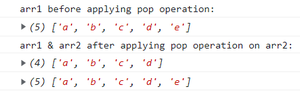3. It can concat a string or merge two or more arrays without using concat() function.

Example:

## JavaScript

 `<``script``>` `    ``// concatenation of two arrays without ` `    ``// concat function using spread syntax` `    ``var breakfast = ["Bread", "Sandwich", "Fruits"];` `    ``var moreBreakfast = ["Salad", "Tea & Coffee"];` `    ``console.log("Breakfast before concatenation:");` `    ``console.log(breakfast);` `    `  `    ``breakfast = [...breakfast, ...moreBreakfast];` `    ``console.log("Breakfast after concatenation:");` `    ``console.log(breakfast);` ``

Output: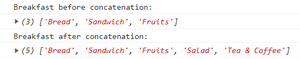4. It allows us to operate Math functions on an array. Because we can’t use Math functions directly on arrays. The spread operator expands an iterable object array into a list of arguments that allow us to operate the Math functions and get the desired result.

Example:

## JavaScript

 `<``script``>` `    ``// Operating an array in an Math` `    ``// function using spread operator` `    ``var arr1 = [99, 50, 130, 1, 98, 23, 66];` `    ``console.log("Maximum element of arr1 is:")` `    ``console.log(Math.max(...arr1));` ``

Output: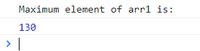5. It allows us to do changes in nested object without reflecting in original object

Example :

Structure of pointing object in nested object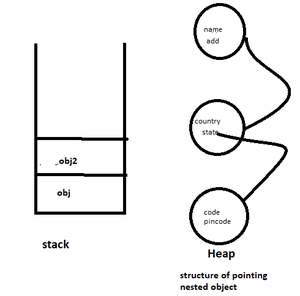## Javascript

 `let obj = {` `   ``name:``'Geeks for Geeks'``,` `   ``add:{` `      ``country:``"INDIA"``,` `      ``state:{` `          ``code:``"DL"``,` `          ``pincode:``"129089"` `      ``}` `  ``}` `}`   `let obj2 = {...obj} ``///SHALLOW COPY` `obj2.name = ``"GFG"``,` `console.log(obj2);`   `obj2 = {...obj2,add:{...obj.add}}` `obj2.add.country = ``"BHARAT"` `console.log(obj2)`   `obj2 = {...obj2,add:{...obj2.add,state:{...obj.add.state}}}  ``//DEEP COPY` `obj2.add.state.pincode  = 823687`   `console.log(``"original object"``)` `console.log(obj);` `console.log(``"doing all changes final object"``)` `console.log(obj2);`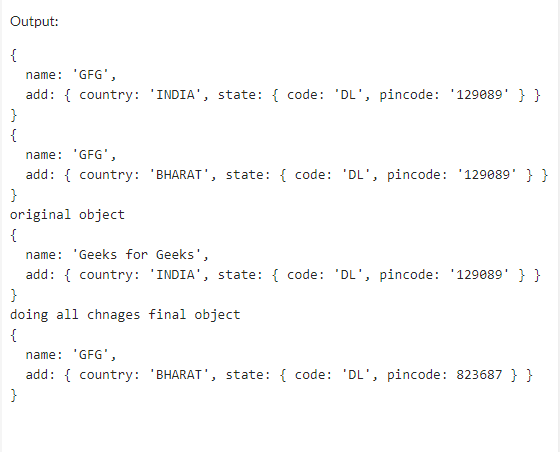Rest operator: The syntax of the Rest operator is similar to that of Spread operator syntax, where the spread operator expands an array into its elements on the other hand rest syntax is used to collect data and store that data into a single variable which we can further pass to a function or can use in our code as a variable.

Syntax:

```function nameOfFunction(...data)
{
// function statement
}```

Example 1: The below example illustrates the use of rest syntax.

## JavaScript

 `<``script``>` `    ``var sum = 0;` `    `  `    ``function Addition(...data) {` `        ``for(var i = 0; i < ``data.length``; i++) {` `            ``sum += data[i];` `        ``}` `        ``console.log("Sum of your passed data is:")` `        ``console.log(sum);` `    ``}` `    ``Addition(95, 98, 2, 51, 6);` ``

Output: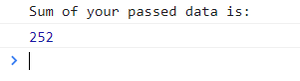Getting the sum of passed data using rest syntax

Example 2:

## JavaScript

 `<``script``>` `    ``var pro = 1;` `    `  `    ``function Multiplication(...param) {` `        ``for(var item = 0; item < ``param.length``; item++) {` `            ``pro *= param[item];` `        ``}` `        ``if(pro < 100) {` `            ``console.log("Product is less than 100:");` `            ``console.log("Product: ", pro);` `            ``pro` `= ``1``;` `            ``console.log("Your new product is: ");` `            ``Multiplication(1, 2, 3, 4, 5);` `            ``console.log("Product: ", pro);` `        ``}` `    ``}` `    ``Multiplication(1, 2, 3, 4);` ``

Output: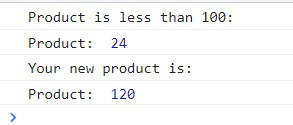Getting the product of passed data using rest syntax

Differences between Rest and Spread Syntax are enclosed in the table below:

Whether you're preparing for your first job interview or aiming to upskill in this ever-evolving tech landscape, GeeksforGeeks Courses are your key to success. We provide top-quality content at affordable prices, all geared towards accelerating your growth in a time-bound manner. Join the millions we've already empowered, and we're here to do the same for you. Don't miss out - check it out now!

Previous
Next